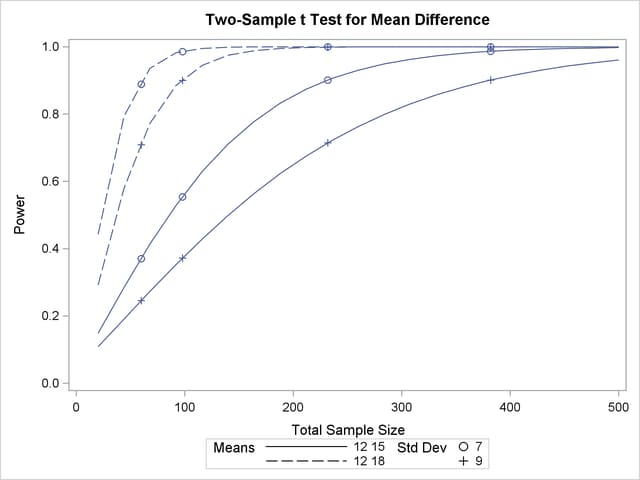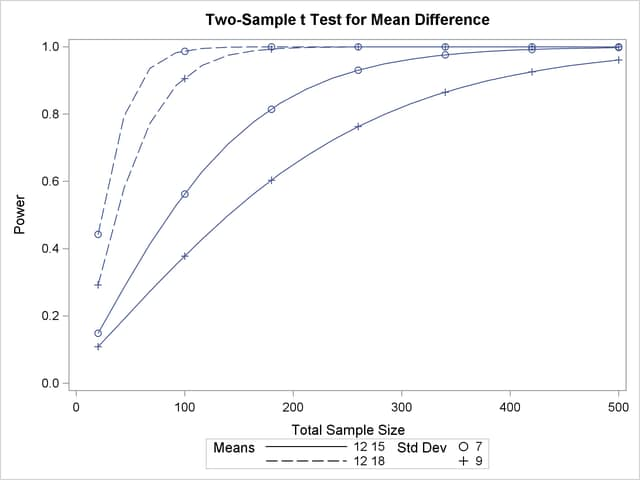### Modifying Symbol Locations

The default locations for plotting symbols are the points computed directly from the power and sample size algorithms. For example, Output 70.8.5 shows plotting symbols corresponding to computed points. The curves connecting these points are interpolated (as indicated by the INTERPOL= option in the PLOT statement).

You can modify the locations of plotting symbols by using the MARKERS= option in the PLOT statement. The MARKERS=ANALYSIS option places plotting symbols at locations corresponding to the input specified in the analysis statement preceding the PLOT statement. You might prefer this as an alternative to using reference lines to highlight specific points. For example, you can reproduce Output 70.8.5, but with the plotting symbols located at the sample sizes shown in Output 70.8.1, by using the following statements:

```proc power plotonly;
twosamplemeans test=diff
groupmeans   = 12 | 15 18
stddev       = 7 9
power        = .
ntotal       = 232 382 60 98;
plot x=n min=20 max=500
markers=analysis;
run;
```

The analysis statement here is the TWOSAMPLEMEANS statement. The MARKERS=ANALYSIS option in the PLOT statement causes the plotting symbols to occur at sample sizes specified by the NTOTAL= option in the TWOSAMPLEMEANS statement: 232, 382, 60, and 98. The resulting plot is shown in Output 70.8.21.

Output 70.8.21 Plot with MARKERS=ANALYSISYou can also use the MARKERS=NICE option to align symbols with the tick marks on one of the axes (the X axis when the X= option is used, or the Y axis when the Y= option is used):

```   plot x=n min=20 max=500
markers=nice;
```

The plot created by this PLOT statement is shown in Output 70.8.22.

Output 70.8.22 Plot with MARKERS=NICENote that the plotting symbols are aligned with the tick marks on the X axis because the X= option is specified.